# CapsNet Trained onMNIST Data

Identify the handwritten digit in an image

Released in 2017, this model makes use of capsules as the fundamental building blocks to replace neurons in artificial neural networks. As opposed to scalar neurons, whose activation reacts to the presence of a particular feature or object, a capsule is a group of neurons all reacting to the same entity, whose vectorial activation can also encode the properties of the detected instance. In addition, features are passed from one capsule layer to another using a novel dynamic routing technique.

Number of layers: 52 | Parameter count: 8,141,840 | Trained size: 33 MB |

## Training Set Information

• MNIST Database of Handwritten Digits, consisting of 60,000 training and 10,000 test grayscale images of size 28x28.

## Performance

• This model achieves 99.5% accuracy on the MNIST dataset.

## Examples

### Resource retrieval

Retrieve the pre-trained net:

 In:=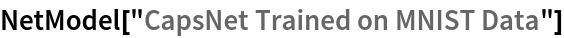Out=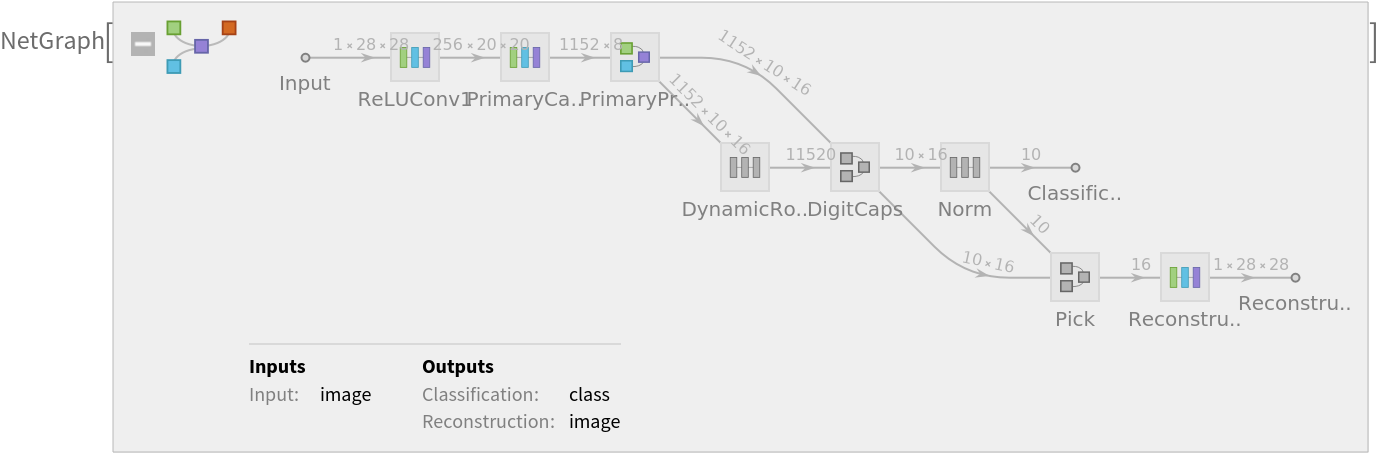### Basic usage

Apply the trained net to a set of inputs:

 In:=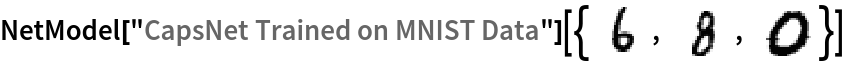Out=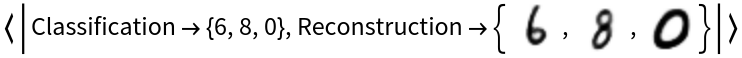Give class probabilities for a single input:

 In:=Out=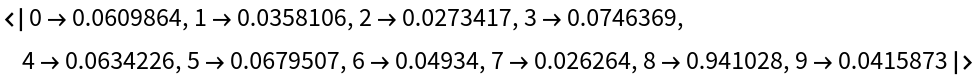### Feature extraction

Create a subset of the MNIST dataset:

 In:=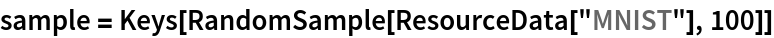Out=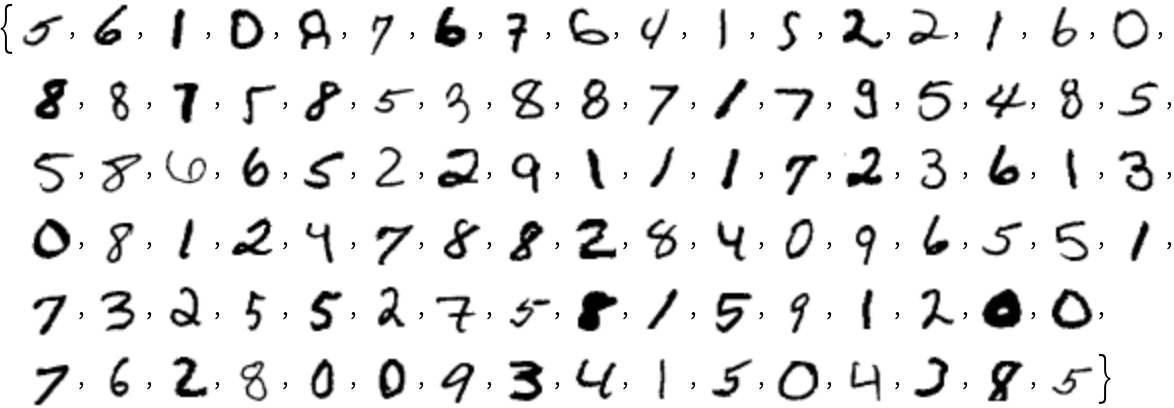Remove the last linear layer of the net, which will be used as a feature extractor:

 In:=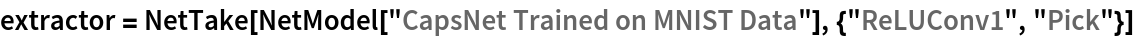Out=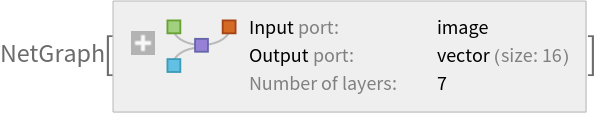Visualize the features of a subset of the MNIST dataset:

 In:=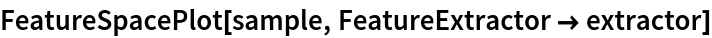Out=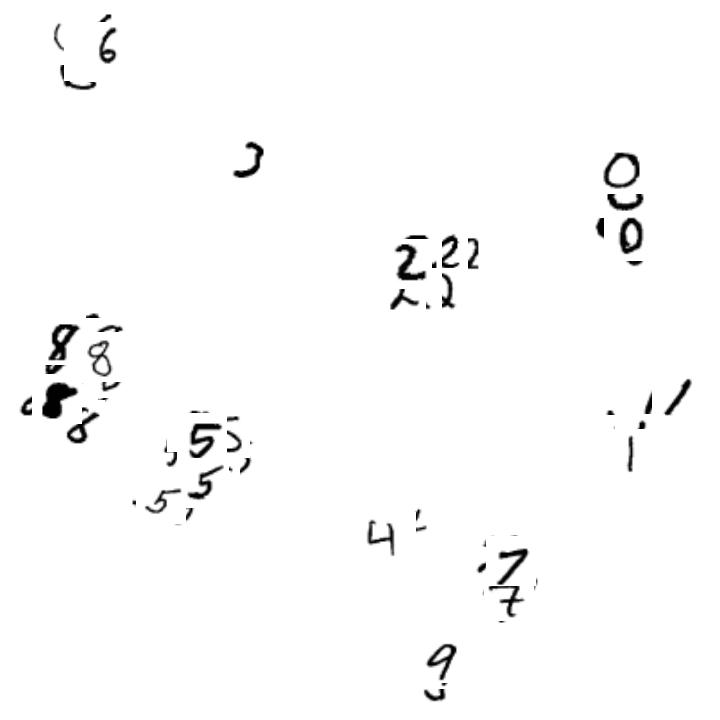### Image generation

Extract the image reconstruction part:

 In:=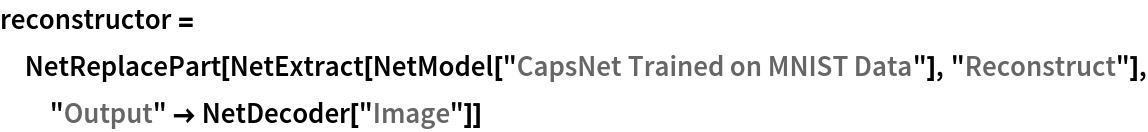Out=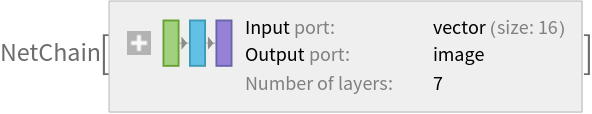Extract the DigitCaps feature vector for a given digit image:

 In:=Out=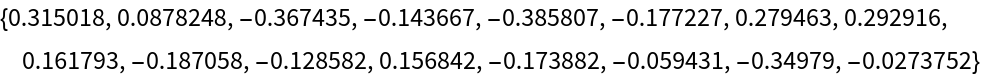Reconstruct the image from the feature vector:

 In:=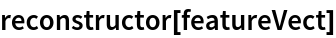Out=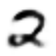Experiment with changing the feature vector. Add a shift along a single coordinate at a time:

 In:=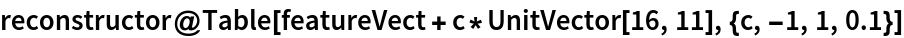Out=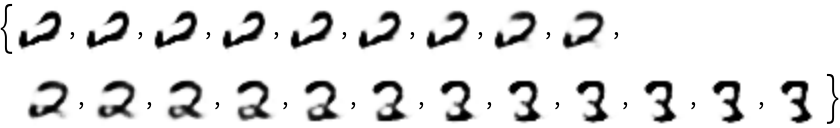In:=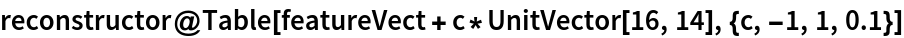Out=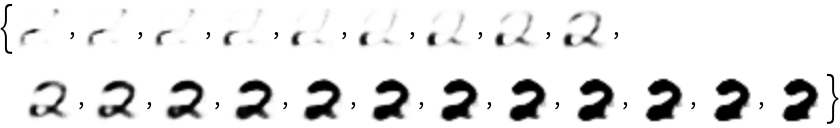In:=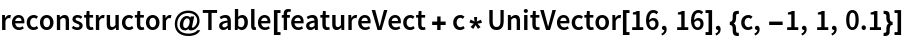Out=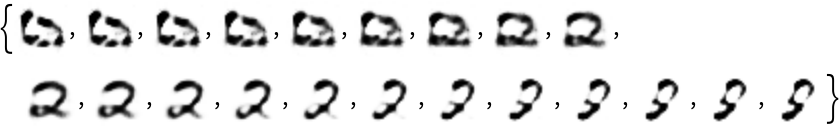### Training the uninitialized architecture

Retrieve the uninitialized training architecture:

 In:=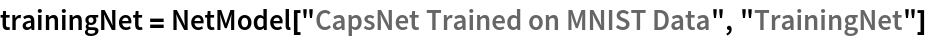Out=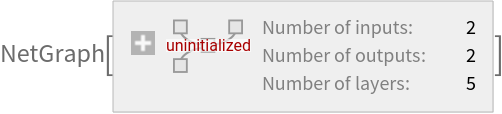Retrieve the MNIST dataset:

 In:=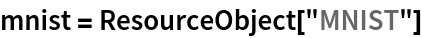Out=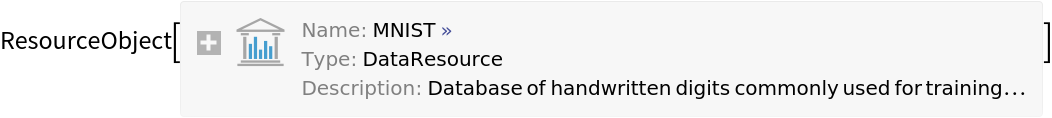Use the training dataset provided:

 In:=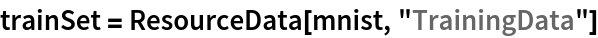Out=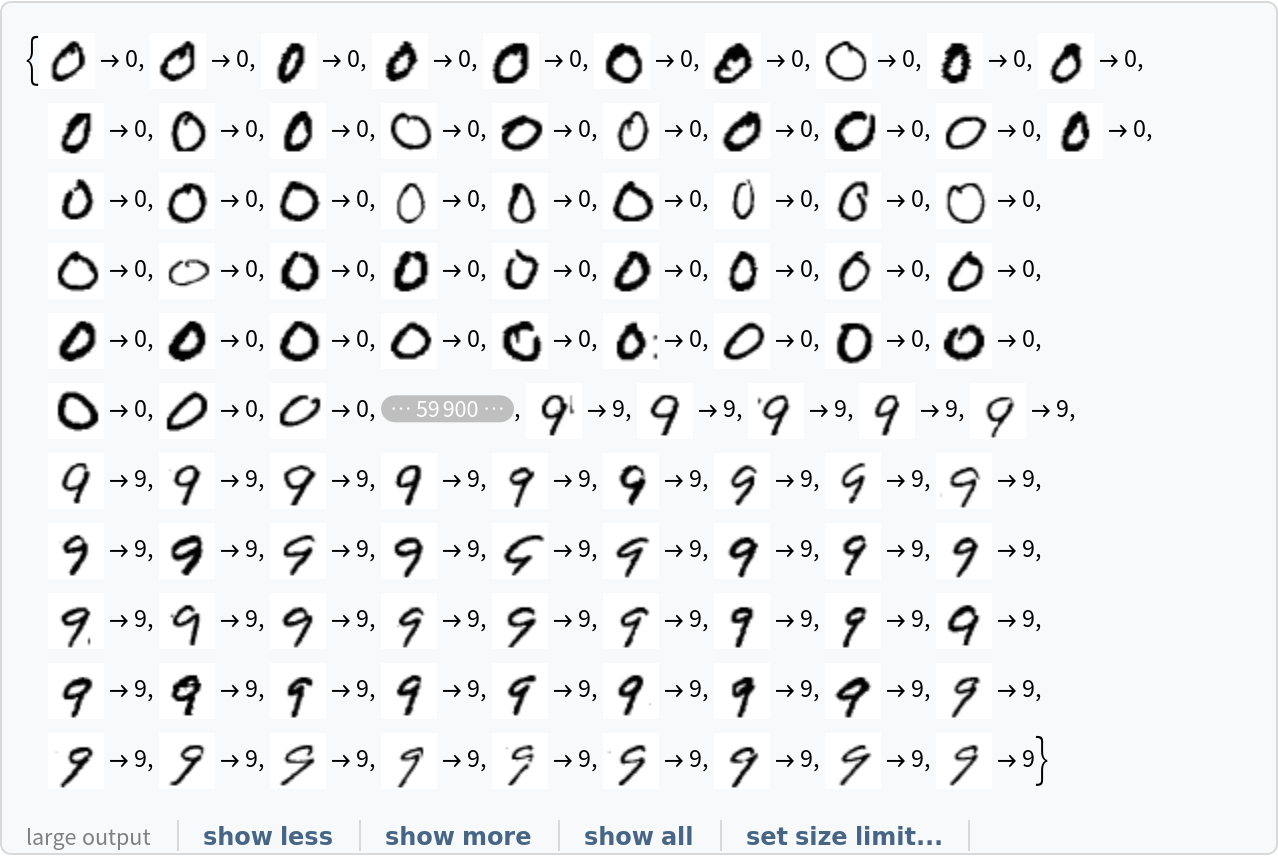Use the test dataset provided:

 In:=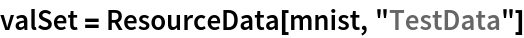Out=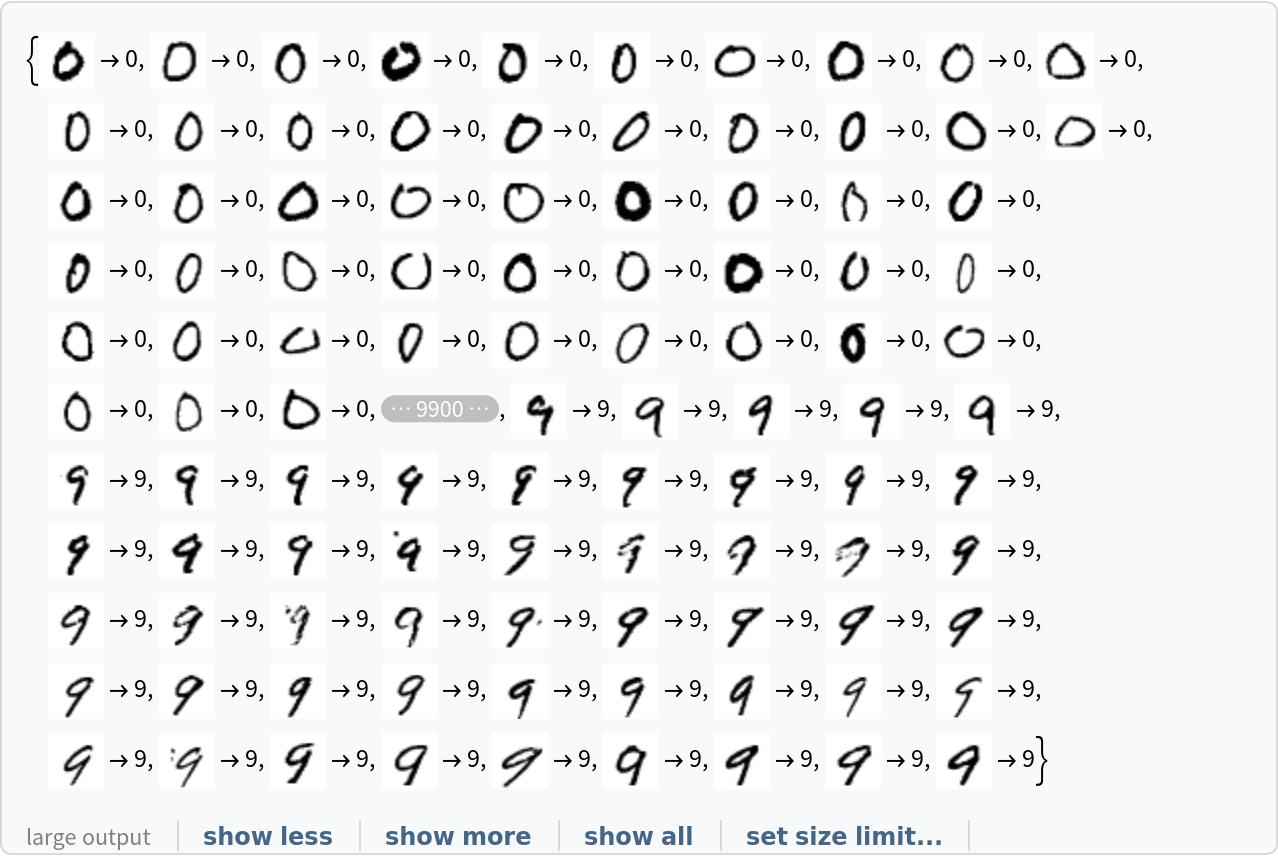Initialize the “W” matrices properly:

 In:=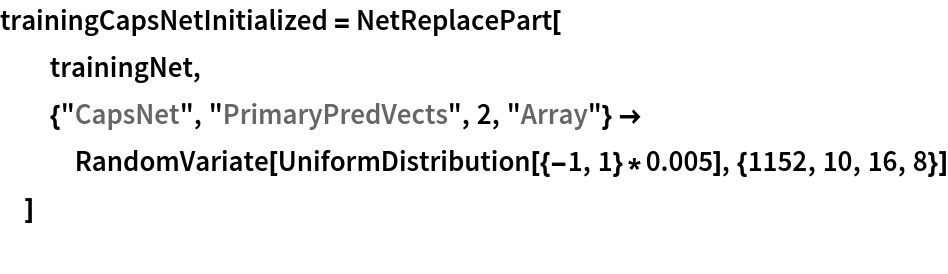Out=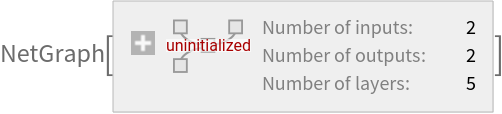Train the net (if a GPU is available, setting TargetDevice -> "GPU" is recommended):

 In:=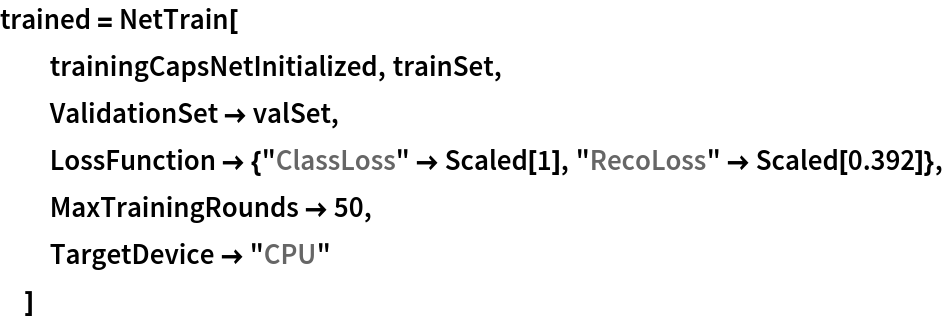Out=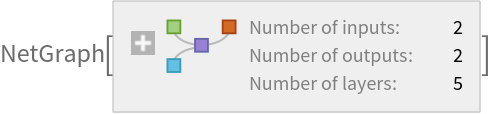### Net information

Inspect the number of parameters of all arrays in the net:

 In:=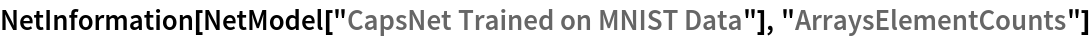Out=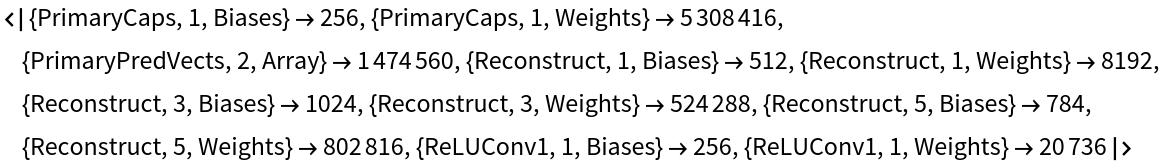Obtain the total number of parameters:

 In:=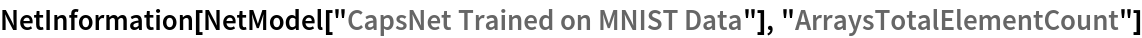Out=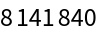Obtain the layer type counts:

 In:=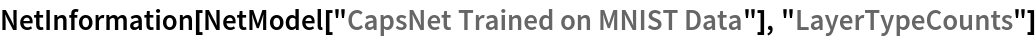Out=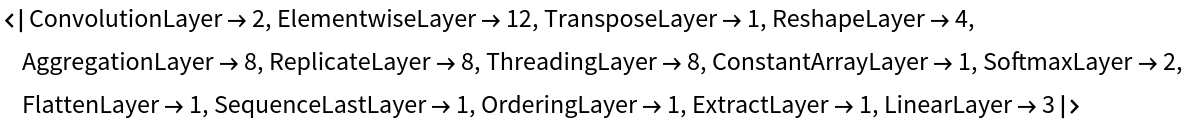Display the summary graphic:

 In:=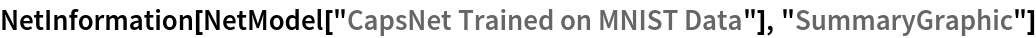Out=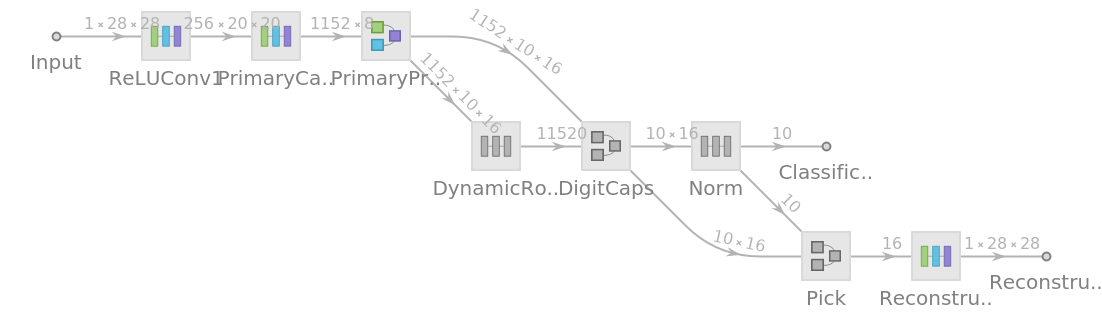### Export to MXNet

Export the net into a format that can be opened in MXNet:

 In:=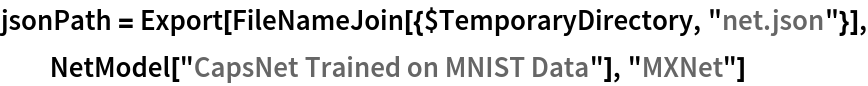Out=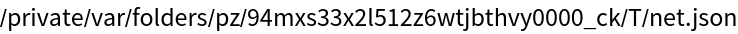Export also creates a net.params file containing parameters:

 In:=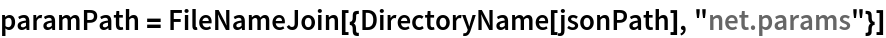Out=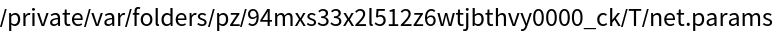Get the size of the parameter file:

 In:=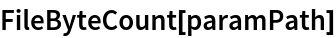Out=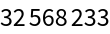The size is similar to the byte count of the resource object:

 In:=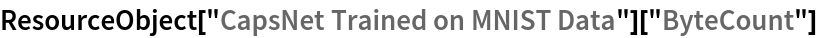Out=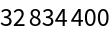Represent the MXNet net as a graph:

 In:=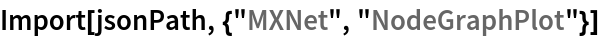Out=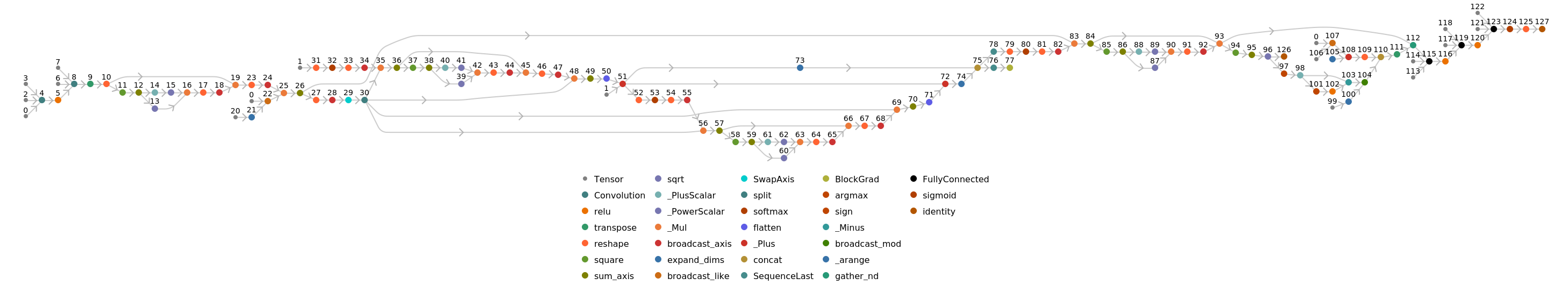## Requirements

Wolfram Language 11.3 (March 2018) or above

## Reference

• S. Sabour, N. Frosst, G. E. Hinton, "Dynamic Routing between Capsules," arXiv:1710.09829 (2017)